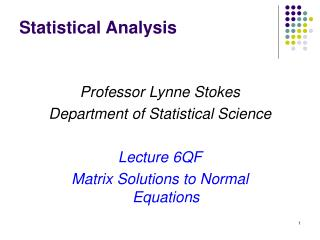DownloadDownload PresentationStatistical Analysis

Statistical Analysis

Télécharger la présentationStatistical Analysis

- - - - - - - - - - - - - - - - - - - - - - - - - - - E N D - - - - - - - - - - - - - - - - - - - - - - - - - - -
Presentation Transcript

1. Statistical Analysis Professor Lynne Stokes Department of Statistical Science Lecture 6QF Matrix Solutions to Normal Equations

2. Direct (Kronecker) Products (Left) Direct Product Some Properties assuming all operations are valid

3. Solving the Normal Equations Single-Factor, Balanced Experiment yij = m + ai + eij i = 1, ..., a; j = 1, ..., r Matrix Formulation y = Xb + e y = (y11 y12 ... y1r ... ya1 ya2 ... yar)’ Show

4. Properties of X’X • Symmetric • Same rank as X • Has an inverse (X’X)-1 iff X has full column rank

5. Solving the Normal Equations Residuals Least Squares Solution: Solve the Normal Equations

6. Solving the Normal Equations Normal Equations Problem: X’X is Singular, has no inverse Show

7. Generalized Inverse: G or A Definition AGA = A not unique Moore-Penrose Generalized Inverse • AGA = A • GAG = G • AG is symmetric • GA is symmetric unique Some Properties if A has full row rank, G = A’(AA’)-1 if A has full column rank, G = (A’A)–1A’ Common Notation A

8. Theorem: For any , X’X X’ = X’ (X’X) (X’X) Solving the Normal Equations Normal Equations Solutions • Every solution to the normal equations corresponds to a generalized inverse of X’X • Every generalized inverse of X’X solves the normal equations

9. A Solution to the Normal Equations One Generalized Inverse Verification

10. A Solution to the Normal Equations Corresponds to the solution to the normal equations with the constraint m = 0 imposed

11. Assignment • Find another generalized inverse for X’X in a one-factor balanced experiment • Verify that it is a generalized inverse • Solve the normal equations using the generalized inverse • Determine what constraint on the model parameters correspond to the generalized inverse